Thursday 30th June 2022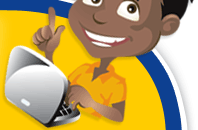# Classroom Key Stage 1 / KS1 - Maths Activities & Resources

## Welcome to the Maths section of the Key Stage 1 classroom. Within KS1 Maths you will find resources, activities and online @school assessment tests that will help children revise and develop the subject of Maths at Key Stage 1 level

•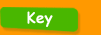•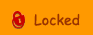•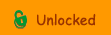••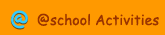•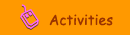•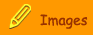###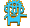Number & The Number System

Dienes Blocks - Interactive test about Tens and Units.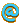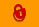Dienes Blocks - Interactive activity where you need to use Dienes blocks to represent numbers.Even Numbers - Test about odd and even numbers.Number - Interactive test about finding odd numbers.Number 1 - Fill in the missing number for this interactive sum activity.Number 2 - Work out what the highest number is on the number grid with this test.Number Circles - Online test to find two numbers that add up to 14.Number Cross - Interactive test about missing numbers in a number sequence.Number Line - Interactive test to answer various sums using a number line.Number Line 1 - Interactive test to find the missing number from this sequence.Number Order - An online activity to help ordering a set of 2 digit numbers.Number Order 2 - Interactive test about ordering numbers.Number Pattern - A test for recognising number patterns.Number Sequence - Interactive test to fill in two numbers in a number sequence.Number Sort - Interactive test to sort numbers under certain criteria.Odds - An interactive test to find odd numbers.